Problem 3:

The infinite matrixwith entries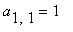,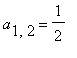,,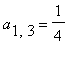,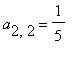,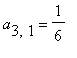, etc., is a bounded operator on.  What is ||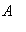||?.

Solution:

The norm is the square root of the largest eigenvalue of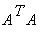, which can be computed by a simple iteration:

Start with a "typical" vector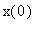, normalized so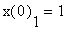, and repeat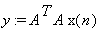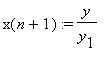Thenconverges to the largest eigenvalue (the second eigenvalue is much smaller, so it converges quite fast).  But we can only work with a finite number of  components, so we truncate to an m by m matrix.  I did it in Maple for up to a 2500 by 2500 matrix (obtaining 1.274224152 5171129126 which happens to have 10 correct digits, but I wasn't confident of the 10th), and Gaston used a C program to get the result for a 32768 by 32768 matrix (of course we don't actually construct the matrix, but work directly with vectors).  His result was 1.274224152 82109, which has 13 correct digits.

Here's the Maple code.

 > mulAA:= proc(V::Array,W::Array,n) # multiply the n-component vector V by A^T*A # W is another vector of the same size used for # intermediate calculations           local i,j;             for i from 1 to n do             W[i]:= add(V[j]/((i+j-1)*(i+j-2)/2+i),j=1..n)           od;           for j from 1 to n do             V[j]:= add(W[i]/((i+j-1)*(i+j-2)/2+i),i=1..n)           od;  end:

 > F:= proc(n) # get the approximate eigenvalue for an n x n # truncation     local V,W,VP,i,eps;     if not type(n,integer) then return 'procname(n)' fi;     eps:= 1.0*10^(-Digits+4);     V:= Array(1..n,datatype=float);     W:= Array(1..n,datatype=float);     V:= 1.0;     for i from 2 to n do V[i]:= 0 od;     do       VP:= copy(V);       mulAA(V,W,n);       if max(seq(abs(V[i]-V*VP[i]),i=1..n)) < eps         then return sqrt(V) fi;       for i from 2 to n do V[i]:= V[i]/V od;       V:= 1.0;     od;     end:

 > Digits:= 15: ti:= time(): F(20); (time()-ti)*seconds; ti:= time(): F(200); (time()-ti)*seconds;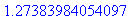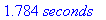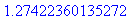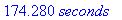Some groups used various extrapolation techniques.  We didn't.

Back to
Solutions to the SIAM \$100 Challenge